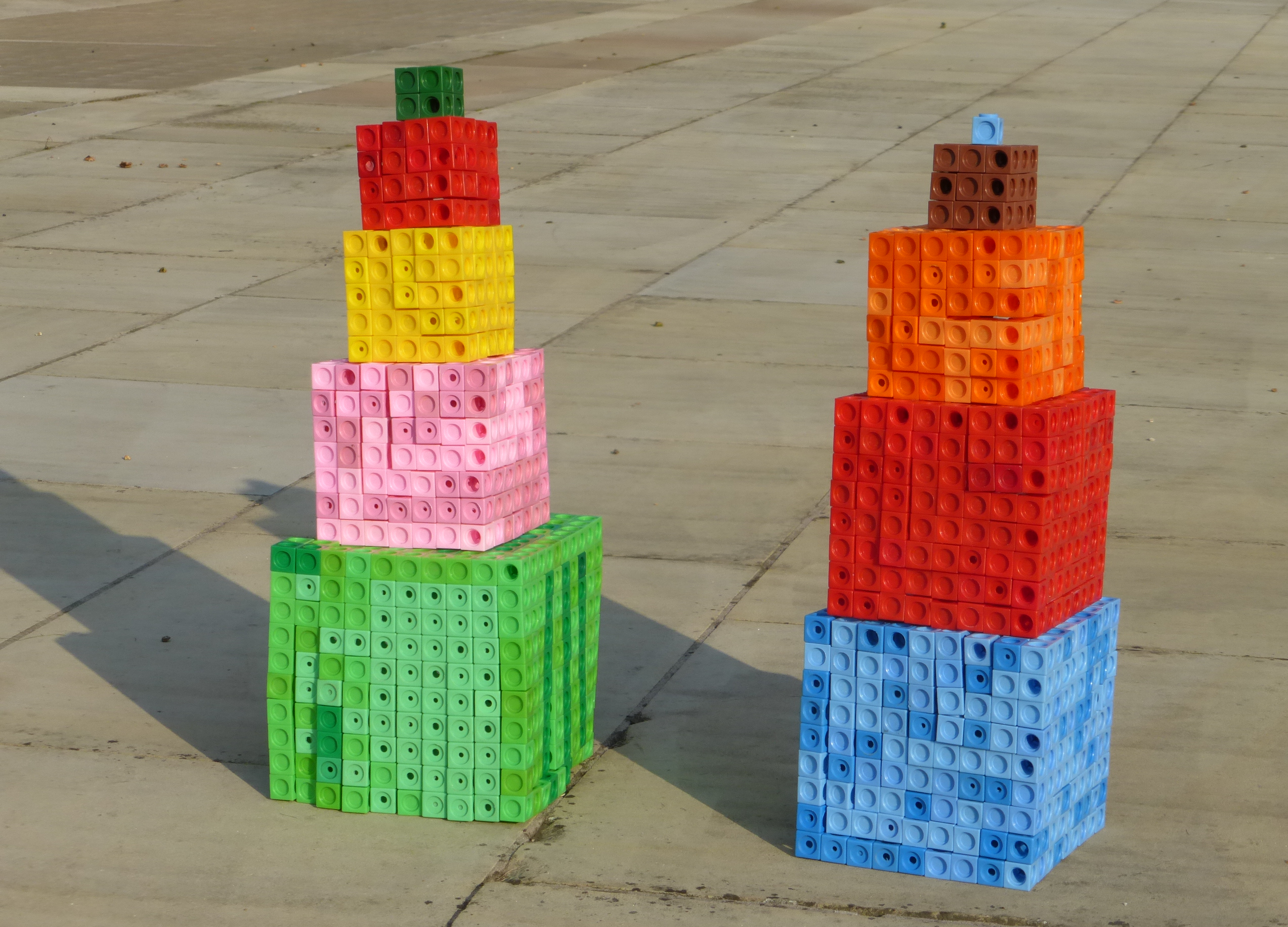#### You may also like### Marbles

I start with a red, a green and a blue marble. I can trade any of my marbles for two others, one of each colour. Can I end up with five more blue marbles than red after a number of such trades?### More Marbles

I start with a red, a blue, a green and a yellow marble. I can trade any of my marbles for three others, one of each colour. Can I end up with exactly two marbles of each colour?### Differences

Can you guarantee that, for any three numbers you choose, the product of their differences will always be an even number?

# Impossibilities

##### Age 11 to 14Challenge LevelSinead has $10$ pockets and $44$ one pound coins.

She wants to put all these pounds into her pockets so that each pocket contains a different number of coins.

Prove that this is impossible.
What is the minimum number of coins Sinead would need in order to be able to do this?Abbie has a set of $10$ plastic cubes, with edges of lengths $1, 2, 3, 4, 5, 6, 7, 8, 9$ and $10$ cm. She tries to build two towers of the same height using all of the cubes.
Prove that this is impossible.

If Abbie has a set of $n$ plastic cubes, with edges of lengths $1$ to $n$, for which values of $n$ can Abbie build two towers of the same height using all of the cubes?

Eustace is adding sets of four consecutive numbers. He wants to find a set where the total is a multiple of $4$.

Prove that this is impossible.

If you enjoyed this challenge, you may wish to try Summing Consecutive Numbers.

With thanks to Don Steward, whose ideas formed the basis of this problem.